# Season’s Greetings Fractal

I don't recall where I found this seasonal fractal. And I can't explain how it works. So please submit a comment if you can shed any light on either of these questions.

### Contents

#### Season's Greetings

I have featured a fractal at this time of the year in two previous years. I see Christmas trees or perhaps a holly decoration.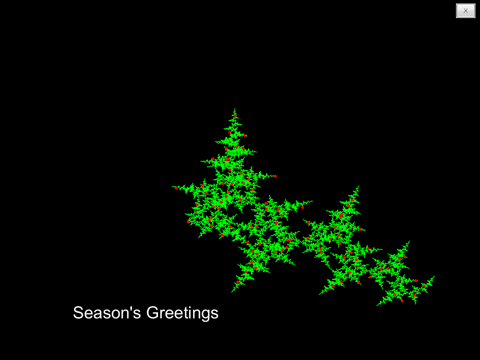#### The Formula

All these figures are obtained by varying the parameters in a formula that generates complex numbers $z$ from the partial sums.

$$z = \sum_n{\exp{(\phi n^p+\sigma) \pi i}}$$

A vector of points in the complex plane is produced by taking $n$ to be a vector of consecutive integers and using the MATLAB cumulative summation function cumsum to compute the partial sums. There are 8600 points in the figure above and 100,000 points in the figures below.

The default value of the parameter $\phi$ is my old friend the golden ratio.

$$\phi = \frac{1+\sqrt{5}}{2}$$

In previous posts I've taken $\phi$ to be other rational and irrational numbers, but today I am sticking to this value.

The parameter $\sigma$ controls the angular orientation. Taking $\sigma$ near $1/8$ makes the large Christmas tree vertical.

While trying how to understand how this thing works I've varied the power $p$ from its usual value of 2 and taken hundreds of thousands of points. This produces today's pictures. Different values of $p$ produce wildly different results.

For a real variable $x$, the expression $\exp (x \pi i)$ is periodic and lies on the unit circle in the complex plane. So we are plotting the cumulative sum of values taken from around the unit circle. At first glance, this appears to be a complex valued random number generator. But it is a lousy generator because we can see Christmas trees in the output.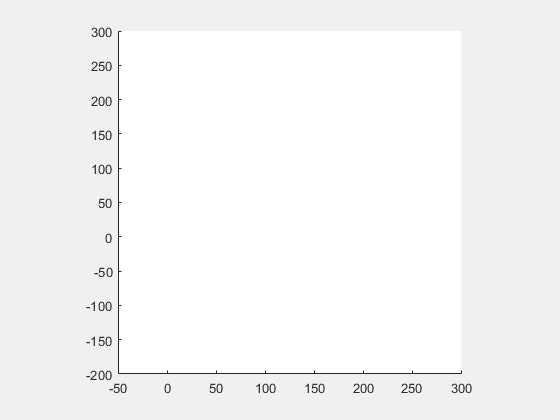#### p = 2/3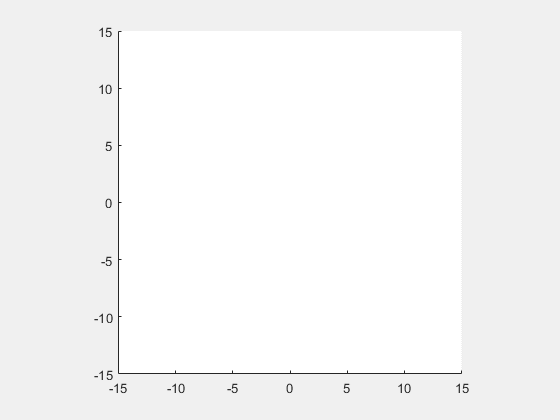#### p = 5/4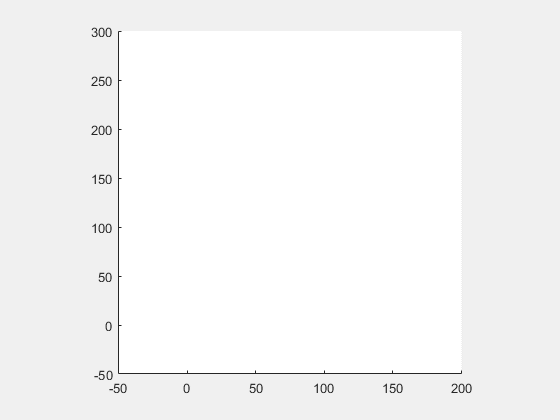#### p = 4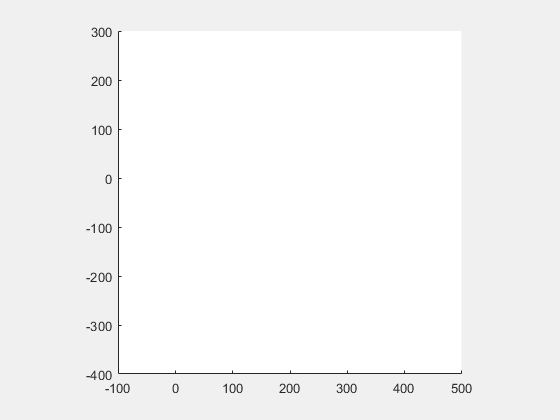#### Today's code

   type greetings_gifs

function greetings_gifs
% Generate animated season's greeting gifs.

% Generate the fractal
phi = (1+sqrt(5))/2;
s = 1/8;
n = 100000;
for gifnum = 1:4
switch gifnum
case 1, p = 2;
case 2, p = 2/3;
case 3, p = 1.25;
case 4, p = 4;
end
w = exp((phi*(0:n).^p+s)*pi*1i);
z = cumsum(w);

% Find local extrema
ks = extrema(z);

% Make an animated gif
plotit(z,p,ks,gifnum)

end % gifnum

% ------------------------

function ks = extrema(z)
n = length(z)-1;
m = n/40;
ks = [];
for j = 0:m:n-m
zj = z(j+1:j+m);
w = zj - mean(zj);
k = find(abs(w) == max(abs(w))) + j;
ks = [ks k];
end
end % extrema

function plotit(z,p,ks,gifnum)
% Make an animated gif
shg
plot(z,'.')
axis square
ax = axis;
gif_frame(['greetings' int2str(gifnum) '.gif'])
clf
axis(ax)
axis square
gif_frame
for j = 1:length(ks)
k = ks(j);
plot(z(1:k),'k.','markersize',0.5)
axis(ax)
axis square
hold on
plot(z(ks(1:j)),'g.','markersize',18)
plot(z(k),'r.','markersize',24)
hold off
title(sprintf('p = %4.2f',p))
gif_frame
end
gif_frame(5)
gif_frame('reset')
end % plotit
end % greetings_gifs


#### Postscript

Happy New Year.

Published with MATLAB® R2016a

|